Question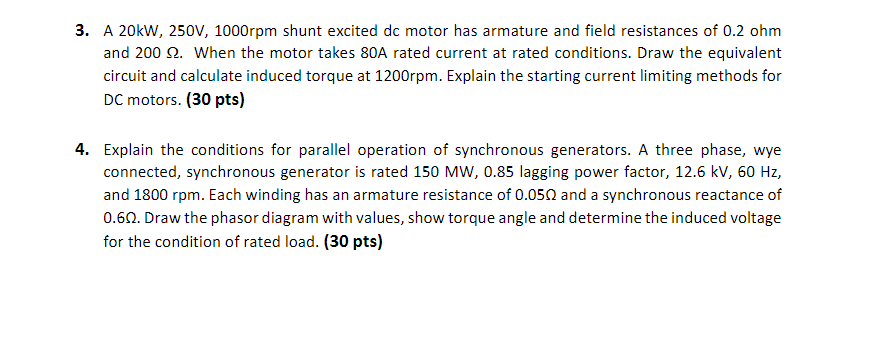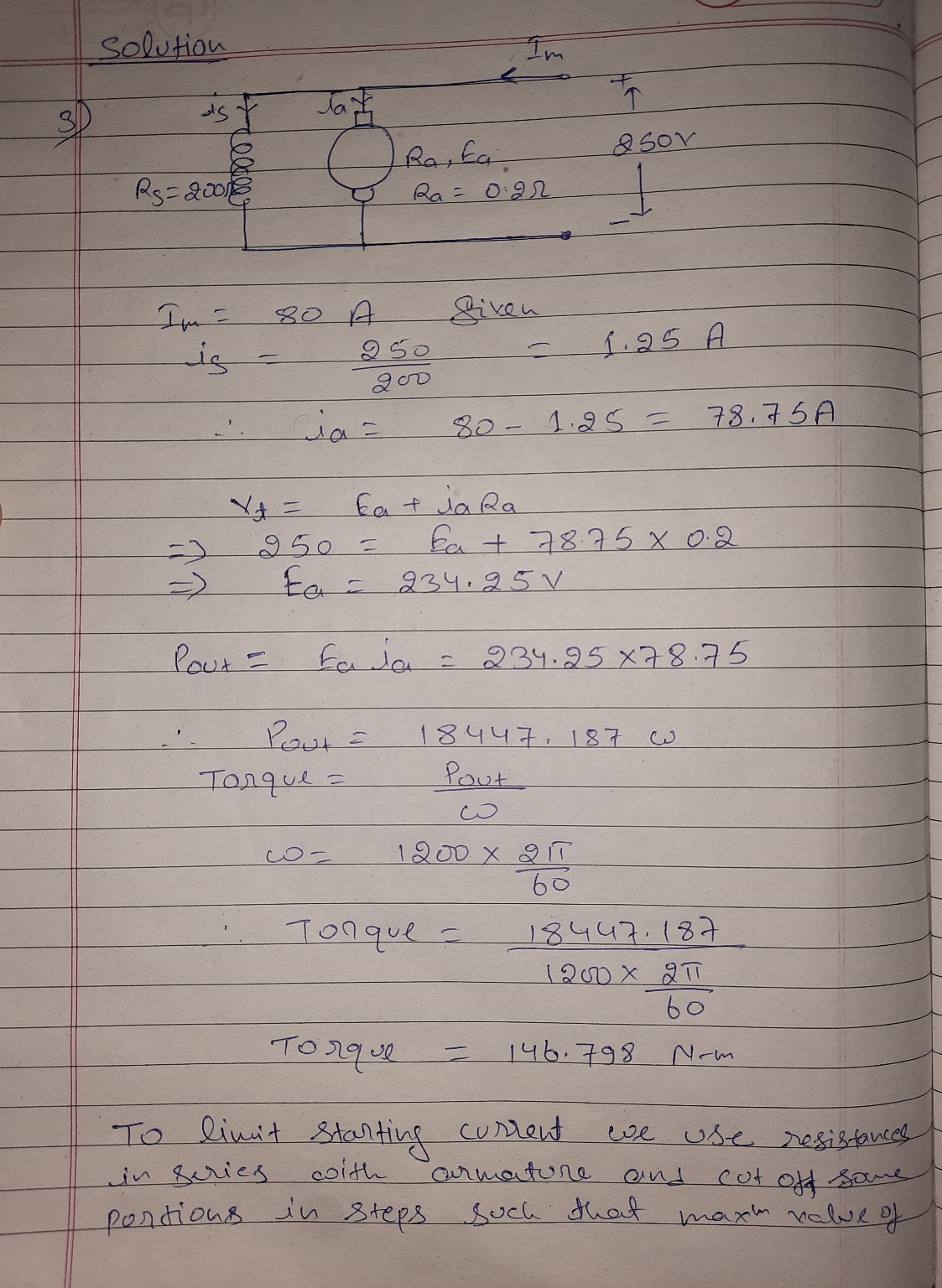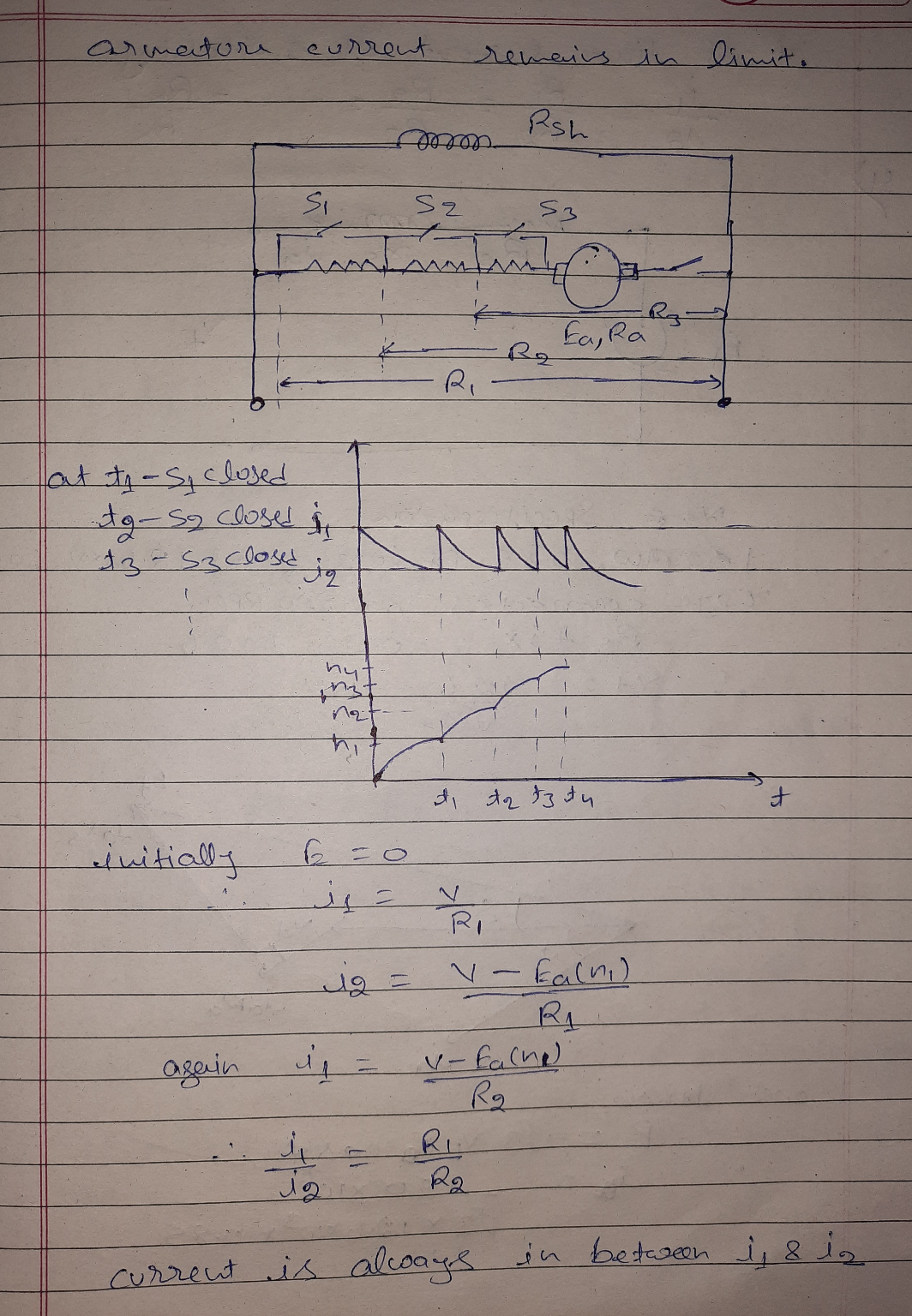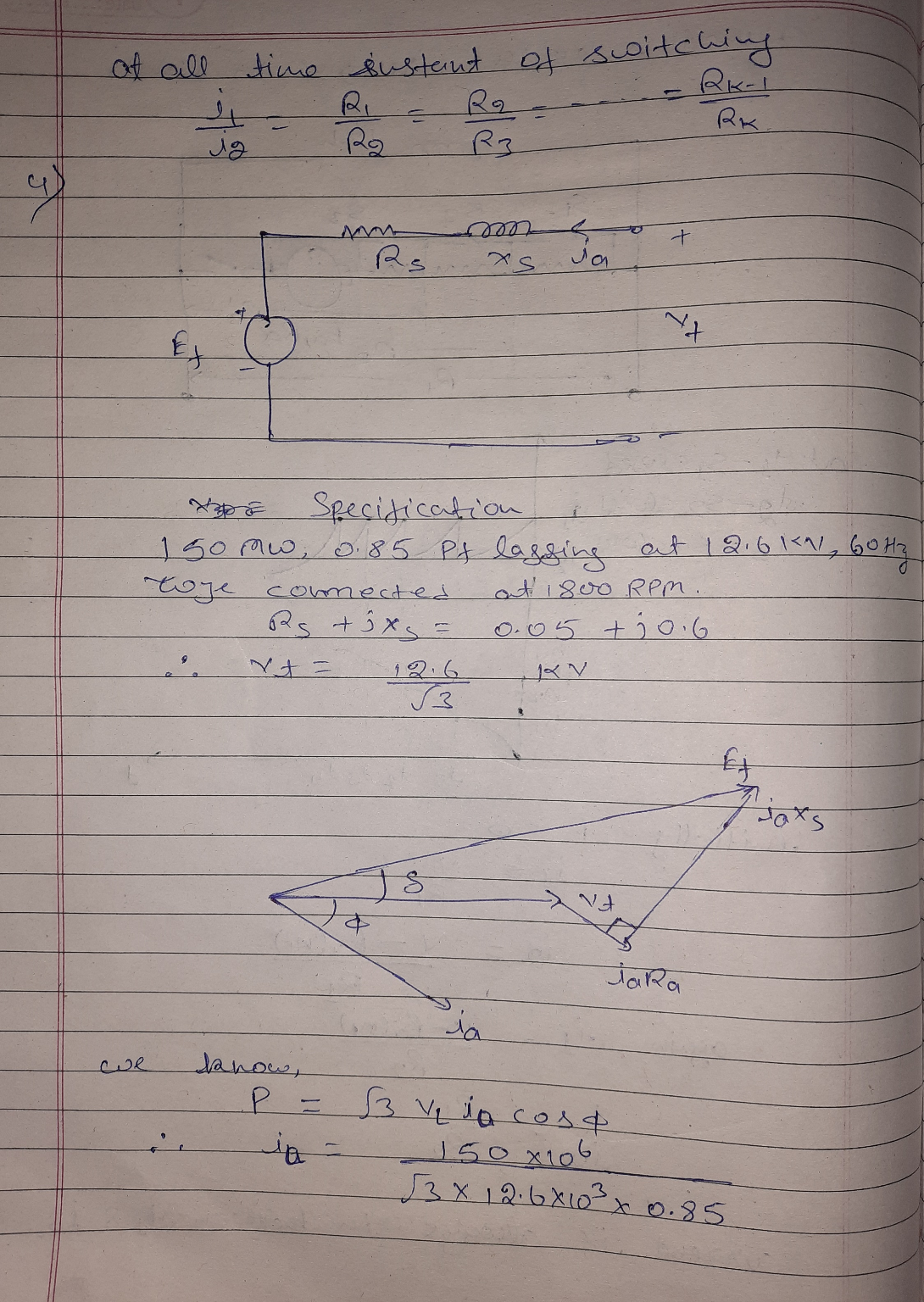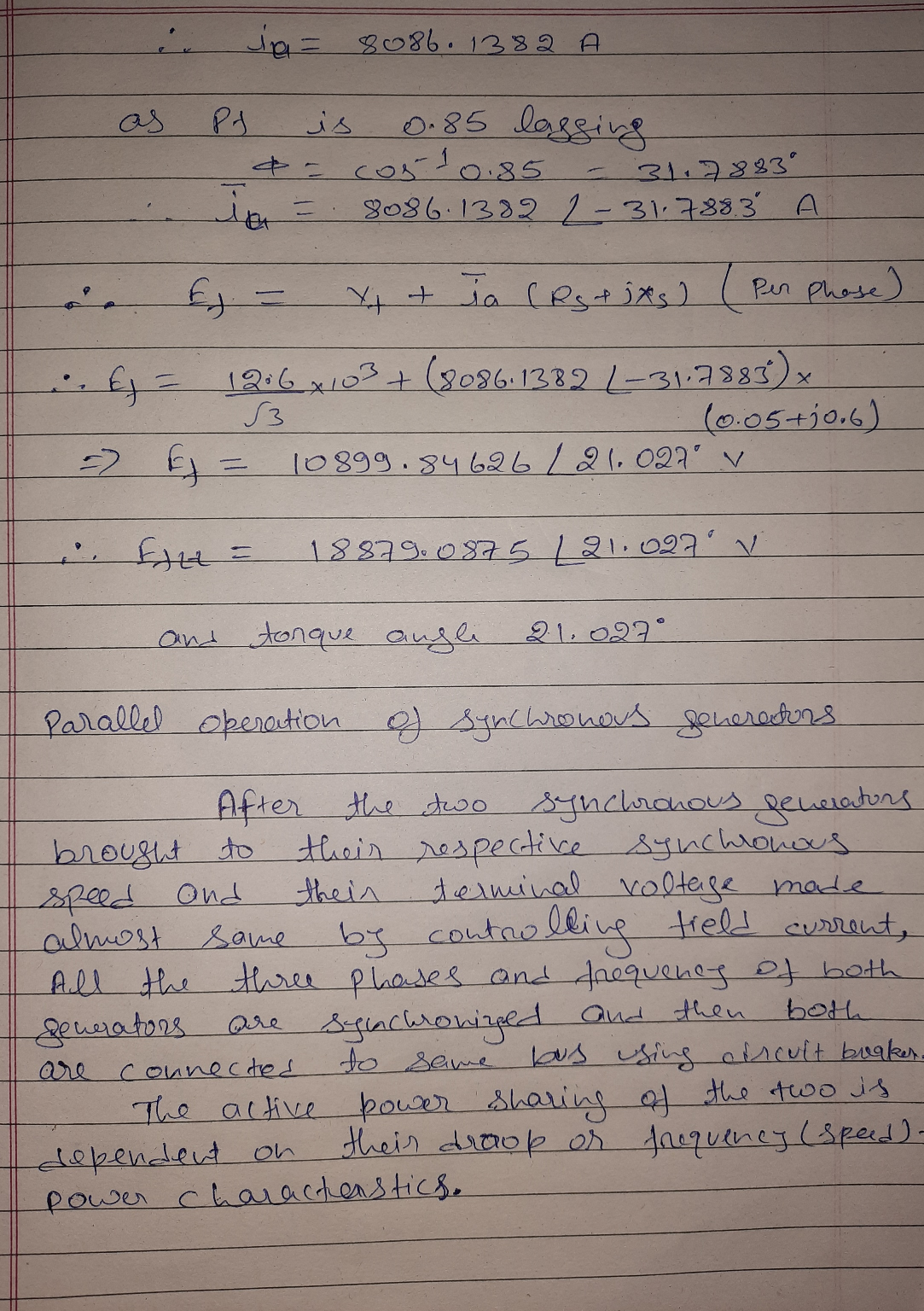#### Earn Coins

Coins can be redeemed for fabulous gifts.

Similar Homework Help Questions
• ### 4. Explain the conditions for parallel operation of synchronous generators. A three phase, wye connected, synchronous...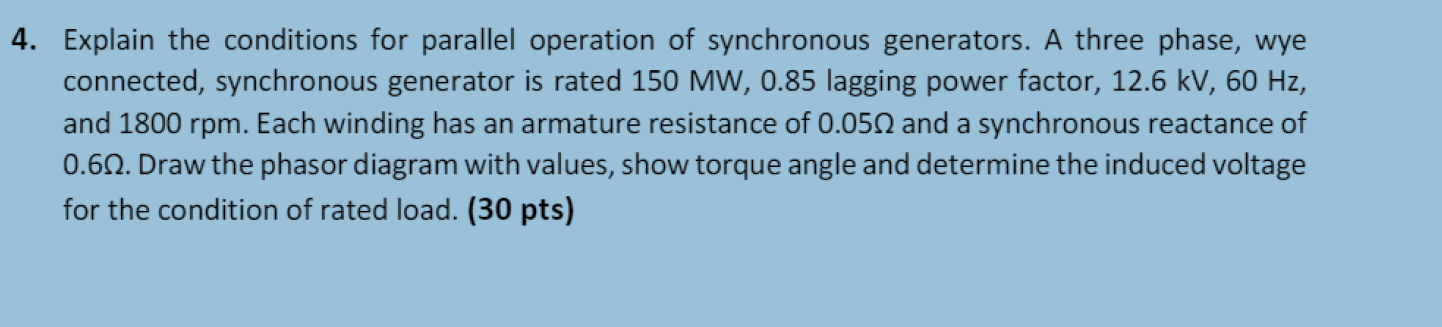4. Explain the conditions for parallel operation of synchronous generators. A three phase, wye connected, synchronous generator is rated 150 MW, 0.85 lagging power factor, 12.6 kV, 60 Hz, and 1800 rpm. Each winding has an armature resistance of 0.0522 and a synchronous reactance of 0.612. Draw the phasor diagram with values, show torque angle and determine the induced voltage for the condition of rated load. (30 pts)

• ### A 250V DC shunt machine has an armature resistance of 0.5Ω and shunt field resistance of...

A 250V DC shunt machine has an armature resistance of 0.5Ω and shunt field resistance of 125Ω, when it is running as a motor, the current taken from the supply is 5A. Calculate the efficiency of the machine i) On no- load ii) As a motor when taking a current of 52A from the supply iii) As a generator when delivering a current of 35A. b) Distinguish between induction motor and synchronous motor.

• ### 3. A 25-kW, 230-V shunt motor has an armature resistance of 0.064 Ω and a field-circuit...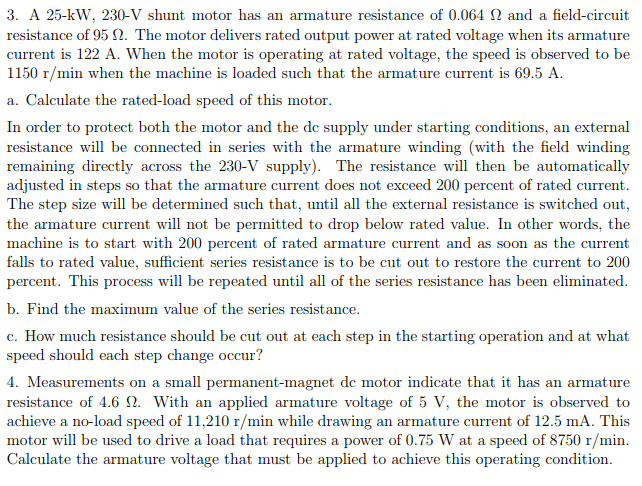3. A 25-kW, 230-V shunt motor has an armature resistance of 0.064 Ω and a field-circuit resistance of 95 Ω. The motor delivers rated output power at rated voltage when its armature current is 122 A. When the motor is operating at rated voltage, the speed is observed to be 1150 r/min when the machine is loaded such that the armature current is 69.5 A a. Calculate the rated-load speed of this motor In order to protect both the motor...

• ### A shunt connected DC motor has independent control over armature and field c The motor ratings...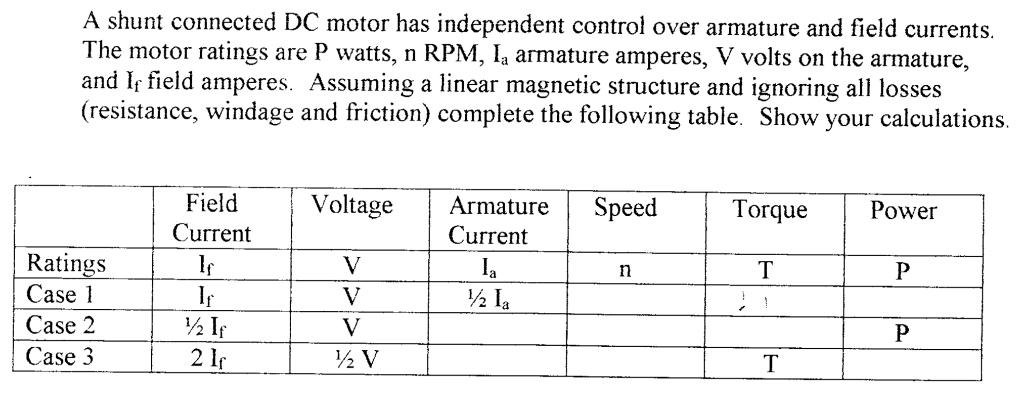A shunt connected DC motor has independent control over armature and field c The motor ratings are P watts, n RPM, Ia armature amperes, V volts on the armature, and Ir field amperes. Assuming a linear magnetic structure and ignoring all losses (resistance, windage and friction) complete the following table. Show your calculations Field Current VoltageArmatureSpeed Current Torque Power Ratings Case l Case 2 Case 3 ½la 2 1r

• ### A 220 V DC shunt motor has an armature resistance, Re-0.1 S2, a field resistance, Ry=100...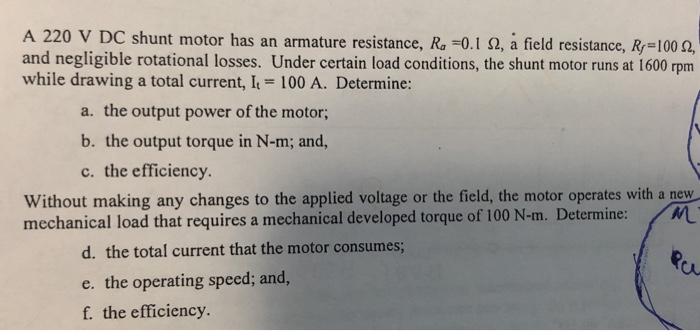A 220 V DC shunt motor has an armature resistance, Re-0.1 S2, a field resistance, Ry=100 12, and negligible rotational losses. Under certain load conditions, the shunt motor runs at 1600 rpm while drawing a total current, I = 100 A. Determine: a. the output power of the motor; b. the output torque in N-m; and, c. the efficiency Without making any changes to the applied voltage or the field, the motor operates with a new M mechanical load that...

• ### A 8250 w shunt DC motor has a terminal voltage of 300 V. A field resistance...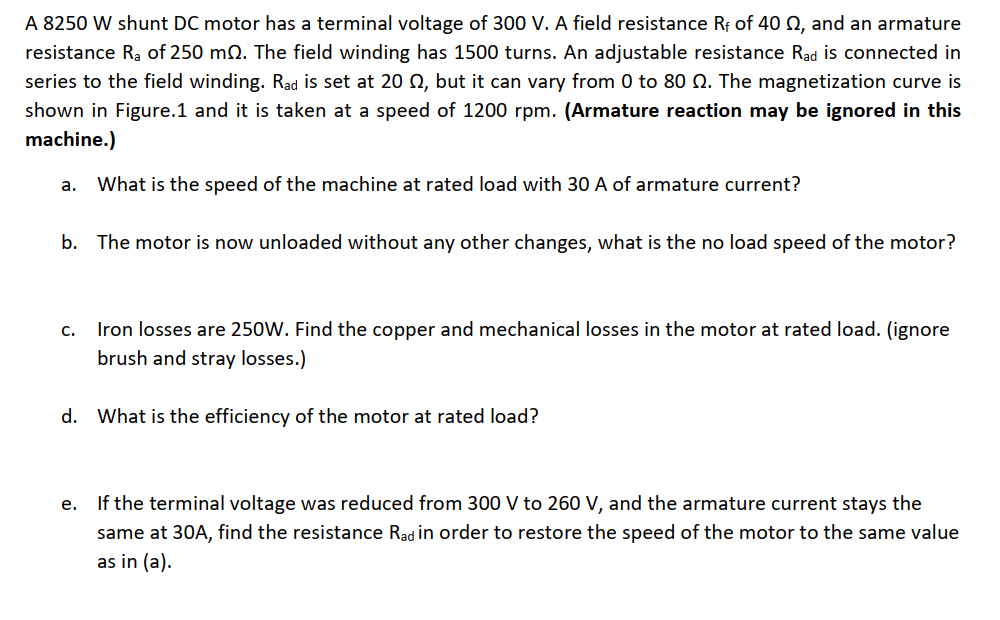A 8250 w shunt DC motor has a terminal voltage of 300 V. A field resistance Rf of 40 Ω, and an armature resistance Ra of 250 mm2. The field winding has 1500 turns. An adjustable resistance Rad is connected in series to the field winding. Rad is set at 20 Ω, but it can vary from 0 to 80 Ω. The magnetization curve is shown in Figure.1 and it is taken at a speed of 1200 rpm. (Armature reaction...

• ### A 30 hp, 240 V, 1150 rpm de shunt motor operating at rated conditions has an efficiency of 88.5%. The armature circuit resistance and field circuit resistance are 0.096 Ω and 936 Ω respectively. Calc...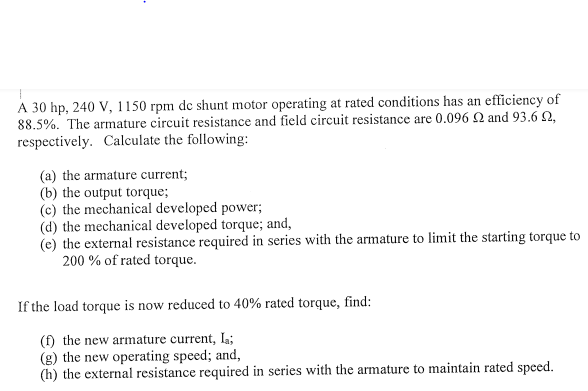A 30 hp, 240 V, 1150 rpm de shunt motor operating at rated conditions has an efficiency of 88.5%. The armature circuit resistance and field circuit resistance are 0.096 Ω and 936 Ω respectively. Calculate the following: (a) the armature current; b) the output torque (c) the mechanical developed power (d) the mechanical developed torque; and, e) the external resistance required in series with the armature to limit the starting torque to 200 % of rated torque. If the load...

• ### Problem 3:A 480 V, 50 kW, 60 Hz, 3-φ synchronous motor has a synchronous reactance of Xs = 4.56 Ω and an armature-to-field mutual inductance, Laf = 150 mH. The motor is operating at rated speed an...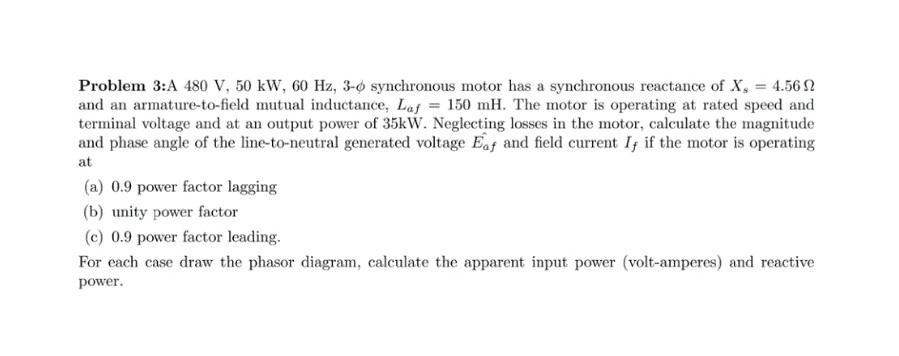Problem 3:A 480 V, 50 kW, 60 Hz, 3-φ synchronous motor has a synchronous reactance of Xs = 4.56 Ω and an armature-to-field mutual inductance, Laf = 150 mH. The motor is operating at rated speed and terminal voltage and at an output power of 35kW. Neglecting losses in the motor, calculate the magnitude and phase angle of the line-to-neutral generated voltage Eaf and field current I if the motor is operating at (a) 0.9 power factor lagging (b) unity...

• ### (i) A 220 volts, 825 rpm, 125 A separately excited DC motor has an armature resistance...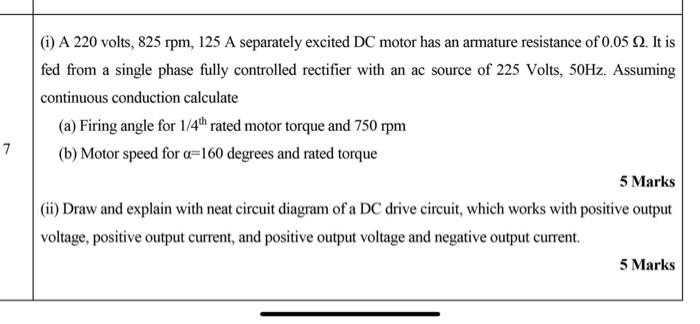(i) A 220 volts, 825 rpm, 125 A separately excited DC motor has an armature resistance of 0.05 2. It is fed from a single phase fully controlled rectifier with an ac source of 225 Volts, 50Hz. Assuming continuous conduction calculate (a) Firing angle for 1/4"hrated motor torque and 750 rpm (b) Motor speed for a=160 degrees and rated torque 5 Marks (ii) Draw and explain with neat circuit diagram of a DC drive circuit, which works with positive output...

• ### b) A 1MW, 3300 V, 50 Hz, wye-connected, 3-ф, twenty four-pole, synchronous motor has a synchronous...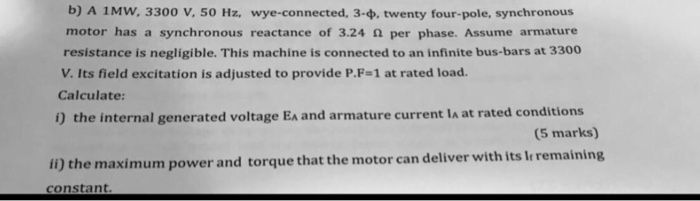b) A 1MW, 3300 V, 50 Hz, wye-connected, 3-ф, twenty four-pole, synchronous motor has a synchronous reactance of 3.24 2 per phase. Assume armature resistance is negligible. This machine is connected to an infinite bus-bars at 3300 V. Its field excitation is adjusted to provide P.F-1 at rated load Calculate: 0 the internal generated voltage EA and armature current la at rated conditions (S marks) ii) the maximum power and torqu e that the motor can deliver with its Ir...